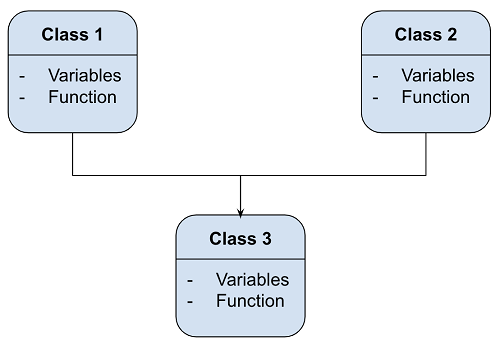# Multiple Inheritance Example in Python

Here, we are going to learn about the Multiple Inheritance in Python and demonstrating the Multiple Inheritance using a Python program.
Submitted by Shivang Yadav, on February 16, 2021

Problem Statement: We will see a program to illustrate the working of multiple inheritance in Python using profit/ loss example.

Problem Description: The program will calculate the net income based on the profits and losses to the person using multiple inheritance.

Multiple Inheritance in Python:

When a single class inherits properties from two different base classes, it is known as multiple inheritance.

The below diagram will make things clearer,All Class and Methods used in the program:

• Class : Profit
• Method : getProfit() -> get input of profit from user.
• Method : printProfit() -> prints profit on screen
• Class : Loss
• Method : getLoss() -> get input of loss from user.
• Method : printLoss() -> prints loss on screen.
• Class : Balance
• Method : getBalance() -> call the getProfit() and getLoss() methods.
• Method : printBalance() -> calls printProfit() and printLoss() methods and calculates balance from profit and loss.

Program to illustrate the Multiple Inheritance in Python

```class Profit:
def getProfit(self):
self._profit=int(input("Enter Profit: "))
def printProfit(self):
print("Profit:",self._profit)

class Loss:
def getLoss(self):
self._loss=int(input("Enter Loss: "))
def printLoss(self):
print("Loss:",self._loss)

class Balance(Profit,Loss):
def getBalance(self):
self.getProfit()
self.getLoss()
self.__balance=self._profit-self._loss
def printBalance(self):
self.printProfit()
self.printLoss()
print("Balance:",self.__balance)

user1 = Balance()
user1.getBalance()
user1.printBalance()
```

Output:

```Enter Profit: 12500
Enter Loss: 7650
Profit: 12500
Loss: 7650
Balance: 4850
```

Preparation

What's New

Top Interview Coding Problems/Challenges!